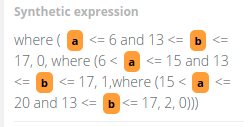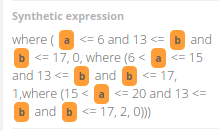# [Solved]Multiple where function

Hello,

I am trying to create a synthetic variable with multiple where functions. How do I do this? I tried the way shown in below, but I don’t think it is working correctly.

Ex)
where ( A <= 6 and 13 <= B <= 17, 0, 0)
where (6< A <= 15 and 13 <= B <= 17, 1, 0)
where (15< A <= 20 and 13 <= B <= 17, 2, 0)

Thank you!

Good day @dongyoun,

Thank you for sharing your question with the community. You need to use the where() expression in a nested way so that the values are valid in all cases, as shown below.

` where ( A <= 6 and 13 <= B <= 17, 0, where (6 < A <= 15 and 13 <= B <= 17, 1,where (15 < A <= 20 and 13 <= B <= 17, 2, 0)))`All the best,
-Isabel

Hi isalogi,

Thank you for the suggestion.

For some reason, the function considers only condition “A” , not condition “B”. Do you know why?

Ex) where ( A <= 6 and 13 <= B <= 17, 1, 0)
In this function, the output should be “1” if A is equal and less than 6 and B is between 13 and 17. When A is less than 6 and B is 30, it still shows “1”. This supposed to be “0”

It would be great if you can give me some suggestions.

Thanks!

Good day @dongyoun,

Thank you for letting me know about this behavior, you are right, that expression outputs a value of one. In this case, it is necessary to make a minor modification to the expression, as shown below.

`where ( A <= 6 and 13 <= B and B <= 17, 0, where (6 < A <= 15 and 13 <= B and B <= 17, 1,where (15 < A <= 20 and 13 <= B and B <= 17, 2, 0)))`With that change, the synthetic variable should be working as expected. Please let me know if you need further assistance.

Best,
-Isabel

Thank you, isalogi.

Is there a limited number of where function can be used?

When I use 4 where functions, it didn’t like it.

Thank you so much for your help.

I am sorry, I found a small mistake. Your suggestion solved the issue.

Thank you!!

1 Like

Hi @dongyoun,